### Home > PC > Chapter 8 > Lesson 8.2.2 > Problem8-89

8-89.
1. Given an, write the first 3 terms of the sequence and then evaluate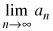. Homework Help ✎

1. an = 6 · (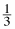)n−1

2. an = −2 · (1.1)n−11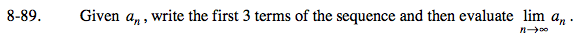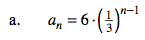$a_1=6\left(\frac{1}{3}\right)^{1-1}=6(1)=6$

$\lim_{x \rightarrow \infty}a_n=6\left(\frac{1}{3}\right)^\infty=0$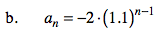$a_1=-2(1.1)^{1-1}=-2$

$\lim_{x \rightarrow \infty}\textit{a}_n=-2(1.1)^\infty=-\infty$